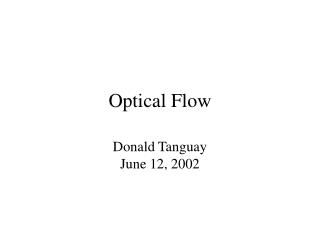DownloadDownload PresentationOptical Flow

# Optical Flow

Download Presentation## Optical Flow

- - - - - - - - - - - - - - - - - - - - - - - - - - - E N D - - - - - - - - - - - - - - - - - - - - - - - - - - -
##### Presentation Transcript

1. Optical Flow Donald TanguayJune 12, 2002

2. Outline • Description of optical flow • General techniques • Specific methods • Horn and Schunck (regularization) • Lucas and Kanade (least squares) • Anandan (correlation) • Fleet and Jepson (phase) • Performance results

3. Optical Flow • Motion field – projection of 3-D velocity field onto image plane • Optical flow – estimation of motion field • Causes for discrepancy: • aperture problem: locally degenerate texture • single motion assumption • temporal aliasing: low frame rate, large motion • spatial aliasing: camera sensor • image noise

4. Brightness Constancy Image intensity is roughly constant over short intervals: Taylor series expansion: Optical flow constraint equation: (a.k.a. BCCE: brightness constancy constraint equation) (a.k.a. image brightness constancy equation)(a.k.a. intensity flow equation)

5. Brightness Constancy

6. Aperture Problem One equation in two unknowns => a line of solutions

7. Aperture Problem In degenerate local regions, only the normal velocity is measurable.

8. Aperture Problem

9. Normal Flow

10. General Techniques • Multiconstraint • Hierarchical • Multiple motions • Temporal refinement • Confidence measures

11. General Techniques • Multiconstraint • over-constrained system of linear equations for the velocity at a single image point • least squares, total least squares solutions • Hierarchical • coarse to fine • help deal with large motions, sampling problems • image warping helps registration at diff. scales

12. Multiple Motions • Typically caused by occlusion • Motion discontinuity violates smoothness, differentiability assumptions • Approaches • line processes to model motion discontinuities • “oriented smoothness” constraint • mixed velocity distributions

13. Temporal Refinement • Benefits: • accuracy improved by temporal integration • efficient incremental update methods • ability to adapt to discontinuous optical flow • Approaches: • temporal continuity to predict velocities • Kalman filter to reduce uncertainty of estimates • low-pass recursive filters

14. Confidence Measures • Determine unreliable velocity estimates • Yield sparser velocity field • Examples: • condition number • Gaussian curvature (determinant of Hessian) • magnitude of local image gradient

15. Specific Methods • Intensity-based differential • Horn and Schunck • Lucas and Kanade • Region-based matching (stereo-like) • Anandan • Frequency-based • Fleet and Jepson

16. Horn and Schunck Minimize the error functional over domain D: smoothnessterm BCCE smoothnessinfluenceparameter Solve for velocity by iterating over Gauss-Seidel equations:

17. Horn and Schunck • Assumptions • brightness constancy • neighboring velocities are nearly identical • Properties + incorporates global information + image first derivatives only • iterative • smoothes across motion boundaries

18. Lucas and Kanade Minimize error via weighted least squares: which has a solution of the form:

20. Lucas and Kanade • Assumptions • locally constant velocity • Properties + closed form solution - estimation across motion boundaries

21. Anandan • Laplacian pyramid – allows large displacements, enhances edges • Coarse-to-fine SSD matching strategy

22. Anandan • Assumptions • displacements are integer values • Properties + hierarchical + no need to calculate derivatives • gross errors arise from aliasing - inability to handle subpixel motion

23. Fleet and Jepson A phase-based differential technique. Complex-valued band-pass filters: Velocity normal to level phase contours: Phase derivatives:

24. Fleet and Jepson • Properties: + single scale gives good results - instabilities at phase singularities must be detected

25. Image Data Sets

26. Image Data Sets • SRI sequence: Camera translates to the right; large amount of occlusion; image velocities as large as 2 pixels/frame. • NASA sequence: Camera moves towards Coke can; image velocities are typically less than one pixel/frame. • Rotating Rubik cube: Cube rotates counter-clockwise on turntable; velocities from 0.2 to 2.0 pixels/frame. • Hamburg taxi: Four moving objects – taxi, car, van, and pedestrian at 1.0, 3.0, 3.0, 0.3 pixels/frame

27. Results: Horn-Schunck